# Explain The Square Law Demodulation and Envelope Demodulation of AM Wave

## Detection or Demodulation of AM Wave

The process of recovering the message signal from the received modulated signal is known as demodulation . This process of detection is exactly opposite to that of modulation .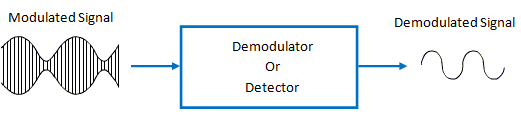Fig 1 : Process of Demodulation or Detection

## Types of Demodulation

There are two types of AM detectors or demodulators such as :

1. Square Law Demodulation
2. Envelope Demodulation

## Square Law Demodulation

The block diagram of square law demodulator is shown in fig 2 .Fig 2: Square Law Demodulator

### Working Operation and Analysis

The input output characteristics i.e., the transfer characteristics of a square law demodulator is non-linear and it is expressed mathematically as :………………(1)

where, v1(t) = input voltage to the detector = AM wave

As we know,Now, substituting for v1(t) in equation (1) , we get……….(2)

But,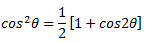Therefore,Substituting this, we get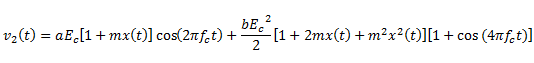Out of these terms, the only desired term is bEcmx(t) which is due to the b v12  term . Hence, the name of this demodulator  is square law demodulator .

This desired term is extracted by using a low pass filter (LPF) after the diode as shown in fig 2 .

Hence, after the LPF, we get

v(t) = ( bEc2m ) x(t) ……………………….(3)

This means that we have recovered the message signal x(t) at the output of the detector .

### Distortion in the Detector Output

Another term which passes through the LPF to the load resistance RL  is  1/2[ bEc2mx(t)] .

This is an unwanted signal and gives rise to a signal distortion .

The ratio of desired signal to the undesired one is given by :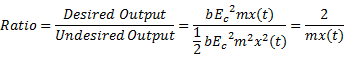This ratio must be maximized in order to minimize the distortion . To achieve this, we should choose |mx(t)| small as compared to unity (1) for all values of t . If m is small, then, the AM wave is weak .

This means that the distortion in the detector output is low if and only if the applied AM is weak and if the percentage modulation is very small .

## Envelope Demodulation

The envelope demodulator is a simple and very efficient device which is suitable for the detection of a narrowband AM signal.

A narrowband AM wave is the one in which the carrier frequency fis much higher as compared to the bandwidth of the modulating signal .

An envelope demodulator produces an output signal that follows the envelope of the input AM signal exactly . It is used in all the commercial AM radio receivers .

### Circuit Diagram

The circuit diagram of the envelope demodulator is shown in fig 3 .Fig 3

The envelope demodulator consists of a diode and RC filter .

### Working Operation

The standard AM wave is applied at the input of the demodulator .

In every positive half cycle of the input, the demodulator diode is forward biased  and charge the filter capacitor C connected across the load resistance R to almost the peak value of the input voltage.

As soon as the capacitor charges to the peak value, the diode stop conducting .

The capacitor will now discharge through R between the positive peaks as shown in fig. 4 . The discharging process continues until the next positive half cycle .

When the input signal becomes greater than the capacitor voltage, the diode conducts again and the process repeats itself .

### Waveforms

The input-output waveforms for the envelope demodulator is shown in fig. 4 .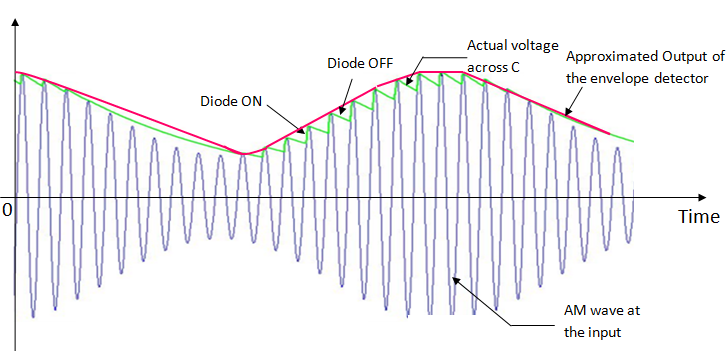Fig 4

It shows the charging  discharging of the filter capacitor and the approximate output voltage .

It may be observed from these waveforms that the envelope of the AM wave is being recovered successfully .

Here we have assumed that the diode is ideal and the AM wave applied to the input of the demodulator is supplied by a source having internal resistance R.

### Selection of the RC time Constants

The capacitor charges through D and Rwhen the diode i on and it discharges through R when the diode is off.

The charging time constant RsC should be short compared to the carrier period 1/f.

Thus,      RsC << 1/fc

On the other hand, the discharging time constant RC should be long enough so that the capacitor discharges slowly through the load resistance R . But, this time constant should not be too long which will not allow the capacitor voltage to discharge at the maximum rate of change of the envelope .

Therefore,             1/f<< RC <<  1/W

where, W = Maximum modulating frequency

### Distortions in the Envelope Demodulator Output

There are two types of distortions which can occur in the detector output such as :

1. Diagonal clipping
2. Negative peak clipping

### Diagonal Clipping

This type of distortion occurs when the RC time constant of the load circuit is too long. Due to this, the RC circuit cannot follow the fast changes in the modulating envelope . The diagonal clipping is shown in fig. 5 .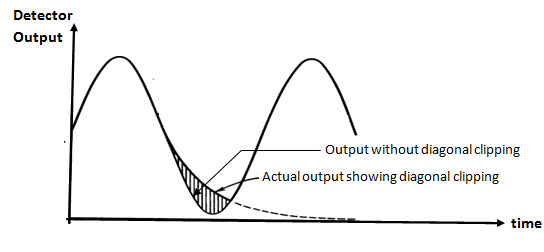Fig 5

### Negative Peak Clipping

This distortion occurs due to a fact that the modulation index on the output side of the detector is higher than that on its input side .

Hence, at higher depth of modulation of the transmitted signal, the overmodulation may takes place at the output of the detector .

The negative peak clipping will take place as a result of this overmodulation as shown in fig 6 .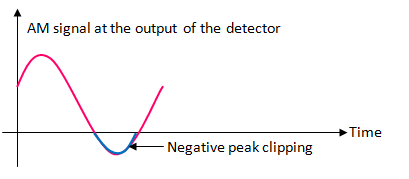Fig 6